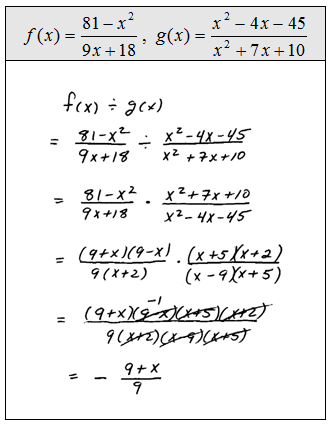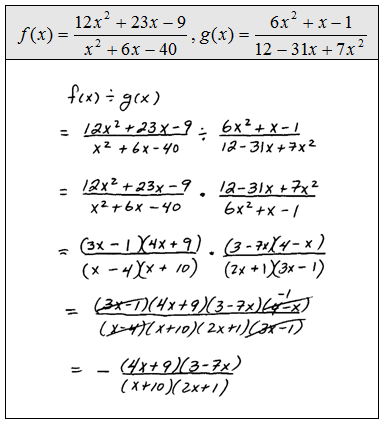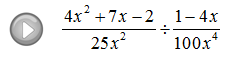## Pages

### Multiplying and Dividing Rational Expressions

Multiplying rational expressions is similar to simplifying them.  We will factor then cancel.

Only cancel factors that are the same, or opposites, in the numerator and denominator.  Recall the property for multiplying fractions,When multiplying fractions there is no need for a common denominator, just multiply the numerators and denominators and then simplify.

Multiply.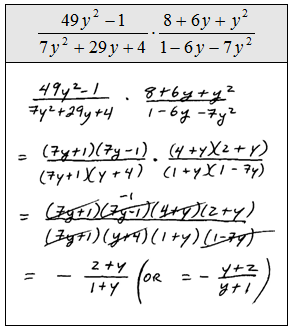The previous questions did not ask for the restrictions but we certainly can list them anyway. Look at the factors in the denominator to see what values for x will evaluate it to zero. Remember that the function notation that implies multiplication.For the given functions find (f *g)(x).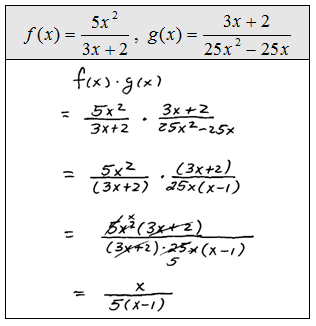When dividing fractions there is no need for a common denominator.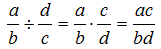However, the above property reminds us that when dividing by a fraction the result will be the same as multiplying by the reciprocal of that fraction. At this point, we will add a step when dividing; we need to reciprocate, factor then cancel.

Divide.The list of restrictions in the previous problem is a bit more involved. As before, look at all the factors in the denominator, even if it was cancelled, to find the values that evaluate to zero.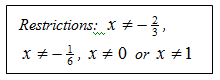Look at the denominators in each step to identify the restrictions.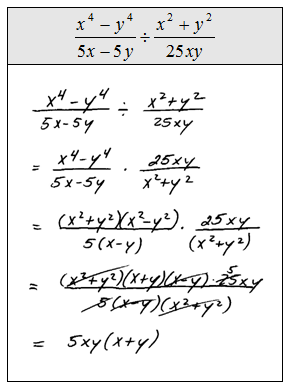Remember that the function notation that implies division.For the given functions find (f / g)(x).